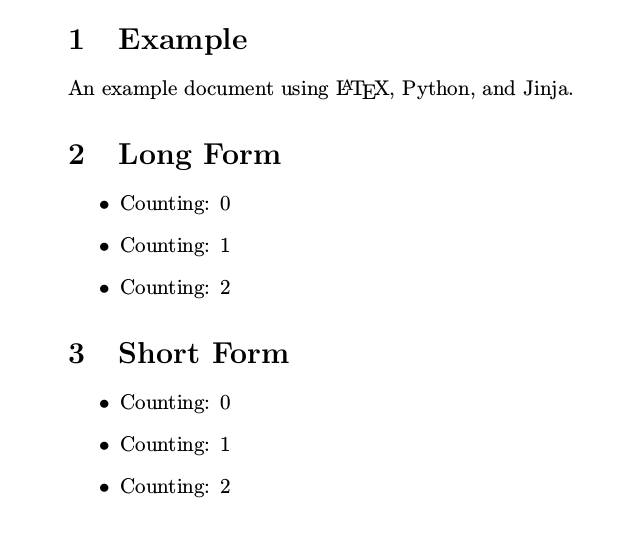The Jinja templating engine works well to create LaTeX templates for generating PDF files. The only issue is the default Jinja block, variable, and comment identification strings can conflict with the LaTeX commands. A solution is to change the Jinja environment to mimic the LaTeX environment.

Here is an example. This is the python file test.py:

import jinja2
import os
from jinja2 import Template
latex_jinja_env = jinja2.Environment(
block_start_string = '\BLOCK{',
block_end_string = '}',
variable_start_string = '\VAR{',
variable_end_string = '}',
comment_start_string = '\#{',
comment_end_string = '}',
line_statement_prefix = '%%',
line_comment_prefix = '%#',
trim_blocks = True,
autoescape = False,
)
template = latex_jinja_env.get_template('jinja-test.tex')
print(template.render(section1='Long Form', section2='Short Form'))


And an example jinja-test.tex to go with it:

\documentclass{article}
\begin{document}
\section{Example}
An example document using \LaTeX, Python, and Jinja.

% This is a regular LaTeX comment
\section{\VAR{section1}}
\#{This is a long-form Jinja comment}
\begin{itemize}
\BLOCK{ for x in range(0, 3) }
\item Counting: \VAR{x}
\BLOCK{ endfor }
\end{itemize}

\section{\VAR{section2}}
%# This is a short-form Jinja comment
\begin{itemize}
%% for x in range(0, 3)
\item Counting: \VAR{x}
%% endfor
\end{itemize}

\end{document}


The above results in test.tex:

\documentclass{article}
\begin{document}
\section{Example}
An example document using \LaTeX, Python, and Jinja.

% This is a regular LaTeX comment
\section{Long Form}
\begin{itemize}
\item Counting: 0
\item Counting: 1
\item Counting: 2
\end{itemize}

\section{Short Form}

\begin{itemize}
\item Counting: 0
\item Counting: 1
\item Counting: 2
\end{itemize}

\end{document}


The resulting PDF:I found harshjv’s Dockerized Texlive 2015 useful for this project.

This is based off an article by Johannes Dollinger](https://web.archive.org/web/20121024021221/http://e6h.de/post/11/") only available via archive.org.